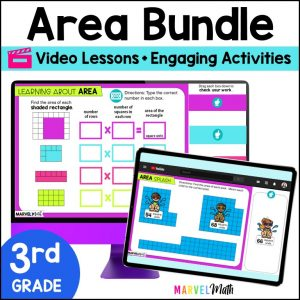Save 10% on your first order with code EXTRA10

# Area of Rectangles using Unit Squares – Digital Activities + Video Lesson

\$4.00\$16.80
SKU: 5396106 Categories: ,

## Description

The fun and engaging Area of Rectangles Practice Activities and Video Lesson are perfect for your lessons, math stations, review or tutoring. This is a great Introduction to Area, connecting students to their understanding of arrays. These Simple and Easy-to-Use Google Slides are designed for 3rd grade students and align to these standards: TEKS 3.6C and CCSS 3.MD.C.6 and 3.MD.C.7.A.

What Teachers are Saying:

⭐⭐⭐⭐⭐ LOVED this resource! The video was perfect for teaching and the activities were easy for the kids to navigate through. Highly recommend! -Nicole T.

⭐⭐⭐⭐⭐ Very thorough resource! I love that it includes a video to help reteach the concepts we are discussing in whole group learning! Plus it is very cute and engaging! -Sara A.

The Video Lesson:

• Teaches students how to use the number of rows of unit squares and the number of unit squares in each row to find area
• Uses a variety of rectangular models
• Walks students through the Google Slides activities they will complete
• Demonstrates how to use the Shape Tool in Google Slides
• Is approximately 8 minutes
• Student Devices must have access to You-Tube to view the Video Lesson

⭐ Many of the slides are self-checking, so students can work through the activity with confidence.

Topics and Activities Include:

• Creating Multiplication Equations to Find Area
• Matching Activities
• Puzzles
• Drawing Rectangles with the Same Area and Different Side Lengths
• Application of Learning in Real-World Word Problems
• Word Problems have optional Step-by-Step support

What’s Included?

• Video Lesson with Activity Instructions
• 13 Interactive Activities
• Teacher Key in separate Google Slides™ doc that can be assigned to students to check their work at home with their parents
• Directions on how to assign the Google Slides™ activity to students in Google Classroom™

Standards Alignment

CCSS.3.MD.C.6 Measure areas by counting unit squares (square cm, square m, square in, square ft, and improvised units).

CCSS.3.MD.C.7.A Find the area of a rectangle with whole-number side lengths by tiling it, and show that the area is the same as would be found by multiplying the side lengths.

TEKS 3.6C Determine the area of rectangles with whole number side lengths in problems using multiplication related to the number of rows times the number of unit squares in each row.

This is Lesson 2 in a comprehensive bundle that covers all the area topics your students need to learn!

Save 30% on this resource by purchasing the Digital Area Bundle!

Lessons in the Digital Area Bundle Include:

Area Lesson 1: Understand the Concept of Area by filling Rectangles with Square Units

Area Lesson 2 *This Resources*: Find Area by Multiplying the Number of Rows by the number of Unit Squares in Each Row

Area Lesson 3: Multiplying Whole Number Side Lengths to find Area

Area Lesson 4: Area of Irregular Shapes using Unit Squares

Area Lesson 5: Area of Irregular Shapes using Side Lengths

Area Lesson 6: Use Area Models to Prove the Distributive Property

You may also be interested in this coordinating Perimeter Bundle.